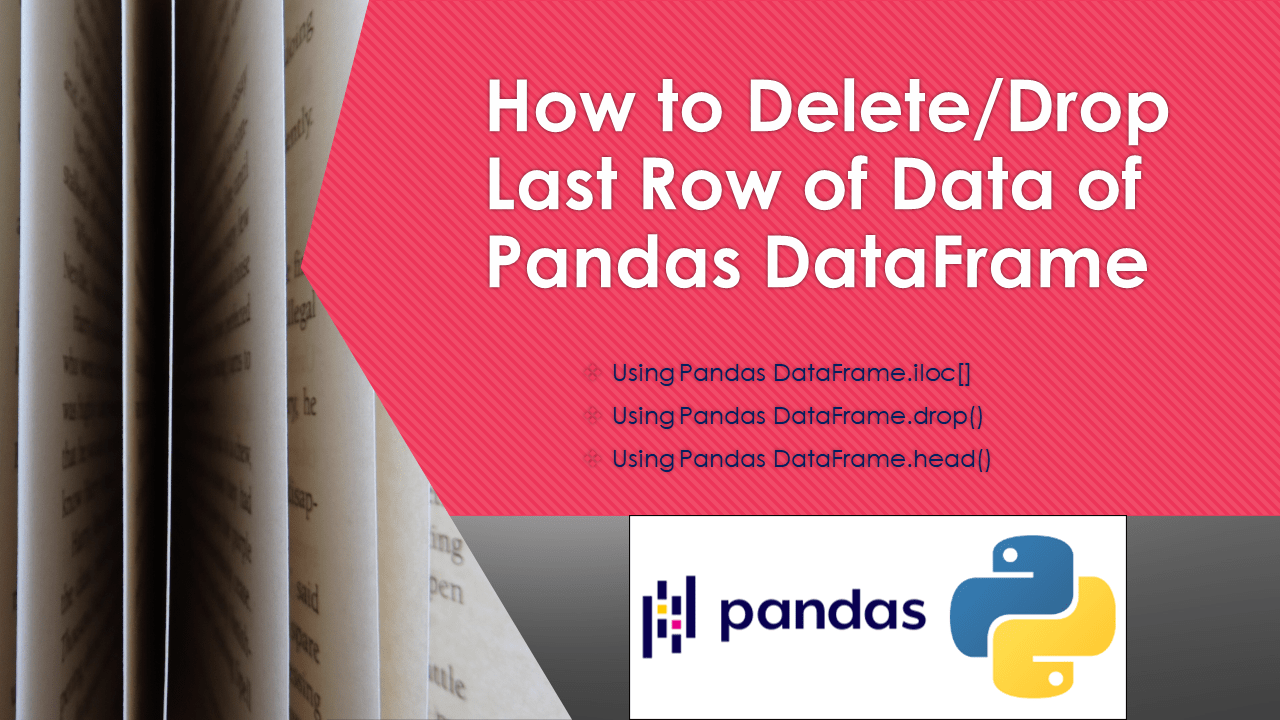# Pandas Delete Last Row From DataFrame

You can delete the last row from the pandas DataFrame using either `drop()`, `iloc[]` and `head()` methods. In this article, I will explain how to delete/drop the last row of data from Pandas DataFrame with examples.

• `drop()` method is used to remove columns or rows from DataFrame;
• Use `axis` param to specify what axis you would like to remove. By default axis = 0 meaning to remove rows. Use axis=1 or columns param to remove columns.
• Use `inplace=True` to remove row/column in place meaning on existing DataFrame with out creating copy.

## 1. Quick Examples of Delete Last Row From Pandas DataFrame

If you are in a hurry, below are some quick examples of how to drop/delete the last row from pandas DataFrame.

``````
# Below are a quick example
# By using iloc[] to select all rows except the last row
df2 = df.iloc[:-1 , :]

# Using drop() function to delete last row
df.drop(index=df.index[-1],axis=0,inplace=True)

# Using DataFrame.head() function to drop last row
``````

Now, let’s create a DataFrame with a few rows and columns and execute some examples and validate results. Our DataFrame contains column names `Courses`,` Fee`, `Duration`, and `Discount`.

``````
import pandas as pd
technologies = {
'Courses':["Spark","PySpark","Python","pandas"],
'Fee' :[20000,25000,22000,24000],
'Duration':['30day','40days','35days','60days'],
'Discount':[1000,2300,2500,2000]
}
index_labels=['r1','r2','r3','r4']
df = pd.DataFrame(technologies,index=index_labels)
print(df)
``````

Yields below output.

``````
Courses    Fee Duration  Discount
r1    Spark  20000    30day      1000
r2  PySpark  25000   40days      2300
r3   Python  22000   35days      2500
r4   pandas  24000   60days      2000
``````

## 2. Drop Last Row of Pandas DataFrame Using iloc[]

By using `DataFrame.iloc[]` you can drop the rows from DataFrame and use `-1` to drop the last row. For example use `df.iloc[:-1,:]` to select all rows except the last one and then assign it back to the original variable which ideally drops the last row from DataFrame.

``````
# By using iloc[] to select all rows except the last row
df2 = df.iloc[:-1 , :]
print(df2)
``````

Yields below output.

``````
Courses    Fee Duration  Discount
r1    Spark  20000    30day      1000
r2  PySpark  25000   40days      2300
r3   Python  22000   35days      2500
``````

## 3. Using drop() Function to Delete Last Row of Pandas DataFrame

Alternatively, you can also use `drop()` method to remove the last row. Use `index` param to specify the last index` `and `inplace=True` to apply the change on the existing DataFrame. In the below example, `df.index[-1]` returns `r3` which is the last row from our DataFrame.

``````
# Using drop() function to delete last row
df.drop(index=df.index[-1],axis=0,inplace=True)
print(df)
``````

Yields same output as above.

## 4. Drop Last Row of Pandas DataFrame Using head() Function

You can also use` df.head(df.shape -1)` to remove the last row of pandas DataFrame.

``````
# Using DataFrame.head() function to drop last row
print(df2)
``````

Yields same output as above.

## 5. Complete Example For Delete Last Row of Data of DataFrame

``````
import pandas as pd
technologies = {
'Courses':["Spark","PySpark","Python","pandas"],
'Fee' :[20000,25000,22000,24000],
'Duration':['30day','40days','35days','60days'],
'Discount':[1000,2300,2500,2000]
}
index_labels=['r1','r2','r3','r4']
df = pd.DataFrame(technologies,index=index_labels)
print(df)

# By using iloc[] to select all rows except the last row
df2 = df.iloc[:-1 , :]
print(df2)

# Using drop() function to delete last row
df.drop(index=df.index[-1],
axis=0,
inplace=True)
print(df)

# Using DataFrame.head() function to drop last row
print(df2)
``````

### Conclusion

In this article, you have learned how to drop the last row data of Pandas DataFrame using `DataFrame.iloc[]`,` DataFrame.drop()`, and `DataFrame.head()` function with examples.

Happy Learning !!

### References